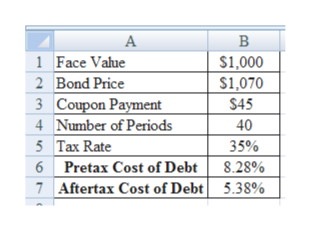# J&R Renovation, Inc., is trying to determine its cost of debt. The firm has a debt issue outstanding with 20 years to maturity that is quoted at 107 percent of face value. The issue makes semiannual payments and has a coupon rate of 9 percent annually. What is the company's pretax cost of debt? (Do not round intermediate calculations. Enter your answer as a percent rounded to 2 decimal places, e.g., 32.16.) Pretax cost of debt             % If the tax rate is 35 percent, what is the aftertax cost of debt? (Do not round intermediate calculations. Enter your answer as a percent rounded to 2 decimal places, e.g., 32.16.) Aftertax cost of debt             %

Question
2 views

J&R Renovation, Inc., is trying to determine its cost of debt. The firm has a debt issue outstanding with 20 years to maturity that is quoted at 107 percent of face value. The issue makes semiannual payments and has a coupon rate of 9 percent annually.

What is the company's pretax cost of debt? (Do not round intermediate calculations. Enter your answer as a percent rounded to 2 decimal places, e.g., 32.16.)

Pretax cost of debt             %

If the tax rate is 35 percent, what is the aftertax cost of debt? (Do not round intermediate calculations. Enter your answer as a percent rounded to 2 decimal places, e.g., 32.16.)

Aftertax cost of debt             %

check_circle

Step 1

Calculation of Pre-tax Cost of Debt and After-tax Cost of Debt:

The pre-tax cost of debt is 8.28% and after-tax cost of debt is 5.38%.

...help_outlineImage TranscriptioncloseВ 1 Face Value \$1,000 \$1,070 2 Bond Price 3 Coupon Payment 4 Number of Periods \$45 40 5 Tax Rate 35% 6 Pretax Cost of Debt 8.28% Aftertax Cost of Debt 7 5.38% fullscreen

### Want to see the full answer?

See Solution

#### Want to see this answer and more?

Solutions are written by subject experts who are available 24/7. Questions are typically answered within 1 hour.*

See Solution
*Response times may vary by subject and question.
Tagged in